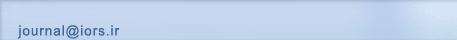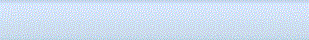[Home ] [Archive]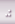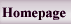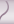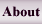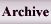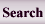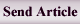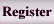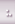Home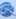Journal InformationArticles archiveSubmission InstructionRegistrationSubmit articleSite FacilitiesContact us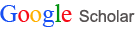All Since 2016 Citations 49076 16489 h-index 9 6 i10-index 9 4

Search in website
 Search the articlesSearch the site Advanced Search
 Enter your Email in the following box to receive the site news and information.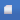Search published articles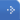Showing 10 results for Subject: Continuous Optimization

A.r. Nazemi, M.h. Farahi,
Volume 4, Issue 2 (10-2013)
Abstract

A high performance numerical technique in the study of aorto-coronaric bypass anastomoses configurations using steady Stokes equations is presented. The problem is first expressed as an optimal control problem. Then, by using an embedding method, the class of admissible shapes is replaced by a class of positive Borel measures. The optimization problem in measure space is then approximated by a linear programming problem. The optimal measure representing optimal shape is approximated by solving this finite-dimensional linear programming problem. An illustrative example demonstrates the effectiveness of the method.
N. Hoseini Monjezi,
Volume 5, Issue 1 (5-2014)
Abstract

Here, a quasi-Newton algorithm for constrained multiobjective optimization is proposed. Under suitable assumptions, global convergence of the algorithm is established.
N Kanzi,
Volume 5, Issue 2 (10-2014)
Abstract

In this paper we study the nonsmooth semi-infinite programming problem with inequality constraints. First, we consider the notions of local cone approximation \$Lambda\$ and \$Lambda\$-subdifferential. Then, we derive the Karush-Kuhn-Tucker optimality conditions under the Abadie and the Guignard constraint qualifications.

Ali Ansari Ardali,
Volume 6, Issue 1 (3-2015)
Abstract

‎In this paper, using the idea of convexificators, we study boundedness and nonemptiness of Lagrange multipliers satisfying the first order necessary conditions. We consider a class of nons- mooth fractional programming problems with equality, inequality constraints and an arbitrary set constraint. Within this context, define generalized Mangasarian-Fromovitz constraint qualification and show that the constraint qualification are necessary and suficient conditions for the Karush- Kuhn-Tucker(KKT) multipliers set to be nonempty and bounded.

Volume 6, Issue 1 (3-2015)
Abstract

‎It is a well-known fact that finding a minimum dominating set and consequently the domination number of a general graph is an NP-complete problem‎. ‎In this paper‎, ‎we first model it as a nonlinear binary optimization problem and then extract two closely related semidefinite relaxations‎. ‎For each of these relaxations‎, ‎different rounding algorithm is exploited to produce a near-optimal dominating set‎. ‎Feasibility of the generated solutions and efficiency of the algorithms are analyzed as well‎.

Dr. Behrouz Kheirfam,
Volume 6, Issue 2 (9-2015)
Abstract

In this paper, we propose an arc-search corrector-predictor
interior-point method for solving \$P_*(kappa)\$-linear
complementarity problems. The proposed algorithm searches the
optimizers along an ellipse that is an approximation of the central
path. The algorithm generates a sequence of iterates in the wide
neighborhood of central path introduced by Ai and Zhang. The
algorithm does not depend on the handicap \$kappa\$ of the problem,
so that it can be used for any \$P_*(kappa)\$-linear complementarity
problem. Based on the ellipse approximation of the central path and
the wide neighborhood, we show that the proposed algorithm has
\$O((1+kappa)sqrt{n}L)\$ iteration complexity, the best-known
iteration complexity obtained so far by any interior-point method
for solving \$P_*(kappa)\$-linear complementarity problems.

Dr. Adil Bagirov, Dr. Sona Taheri,
Volume 8, Issue 2 (5-2017)
Abstract

Clustering problems with the similarity measure defined by the \$𝐿_1\$-norm are studied. Characterizations of different stationary points of these problems are given using their difference of convex representations. An algorithm for finding the Clarke stationary points of the clustering problems is designed and a clustering algorithm is developed based on it. The clustering algorithm finds a center of a data set at the first iteration and gradually adds one cluster center at each consecutive iteration. The proposed algorithm is tested using large real world data sets and compared with other clustering algorithms.
Prof. Ali Farajzadeh, Dr Parisa Cheraghi,
Volume 9, Issue 2 (6-2018)
Abstract

In this paper, we investigate relation between weak subdifferential and augmented normal cone. We define augmented normal cone via weak subdifferential and vice versa. The necessary conditions for the global maximum are also stated. We produce preliminary properties of augmented normal cones and discuss them via the distance function. Then we obtain the augmented normal cone for the indicator function. Relation between weak subifferential and augmented normal cone and epigraph is also explored. We also obtain optimality conditions via weak subdifferential and augmented normal cone. Finally, we define the Stampacchia and Minty solution via weak subdifferential and investigate the relation between Stampacchia and Minty solution and the minimal point.
Mr Saeed Fallahi, Prof. Maziar Salahi, Mr Saeed Ansary Karbasy,
Volume 9, Issue 2 (6-2018)
Abstract

We consider the extended trust region subproblem (eTRS) as the minimization of an indefinite quadratic function subject to the intersection of unit ball with a single linear inequality constraint. Using a variation of the S-Lemma, we derive the necessary and sufficient optimality conditions for eTRS. Then, an OCP/SDP formulation is introduced for the problem. Finally, several illustrative examples are provided.
Dr Zhang Wei, Prof. Cornelis Roos,
Volume 9, Issue 2 (6-2018)
Abstract

We deal with a recently proposed method of Chubanov , for solving linear homogeneous systems with positive variables. We use Nesterovchr('39')s excessive gap method in the basic procedure. As a result, the iteration bound for the basic procedure is reduced by the factor \$nsqrt{n}\$. The price for this improvement is that the iterations are more costly, namely \$O(n^2 )\$ instead of \$O(n)\$. The overall gain in the complexity hence becomes a factor of \$sqrt{n}\$.

 Page 1 from 1